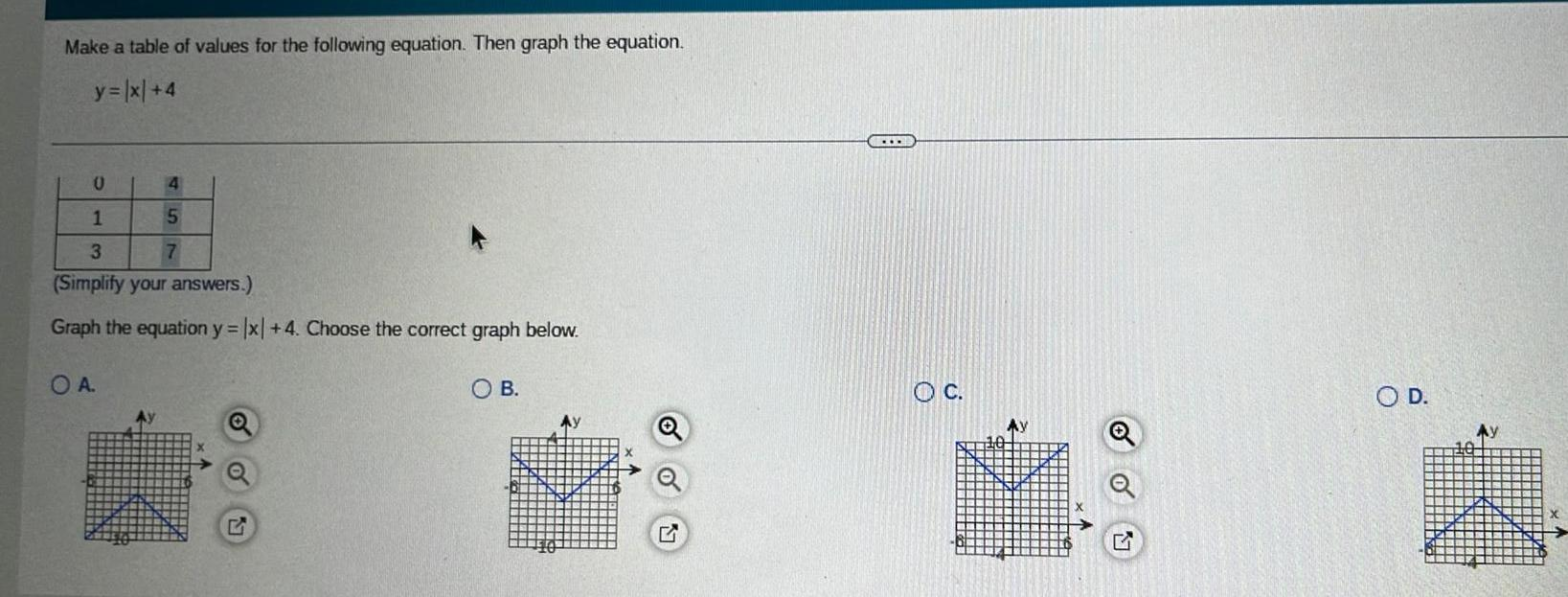Question:

# Make a table of values for the following equation Then graph

Last updated: 9/14/2023Make a table of values for the following equation Then graph the equation y x 4 0 4 1 5 3 7 Simplify your answers Graph the equation y x 4 Choose the correct graph below O A OB G OC O D